1666770774

# Variables in Python

In this Python tutorial for beginners, we learn about Variables in Python. Variables are containers for storing data values. A Python variable is a symbolic name that is a reference or pointer to an object.

## Creating Variables

Python has no command for declaring a variable.

A variable is created the moment you first assign a value to it.

### Example

``````x = 5
y = "John"
print(x)
print(y)``````

Variables do not need to be declared with any particular type, and can even change type after they have been set.

### Example

``````x = 4       # x is of type int
x = "Sally" # x is now of type str
print(x)``````

## Casting

If you want to specify the data type of a variable, this can be done with casting.

### Example

``````x = str(3)    # x will be '3'
y = int(3)    # y will be 3
z = float(3)  # z will be 3.0``````

## Get the Type

You can get the data type of a variable with the `type()` function.

### Example

``````x = 5
y = "John"
print(type(x))
print(type(y))``````

## Single or Double Quotes?

String variables can be declared either by using single or double quotes:

### Example

``````x = "John"
# is the same as
x = 'John'``````

## Case-Sensitive

Variable names are case-sensitive.

### Example

This will create two variables:

``````a = 4
A = "Sally"
#A will not overwrite a``````

## What is a Variable in Python?

A Python variable is a reserved memory location to store values. In other words, a variable in a python program gives data to the computer for processing.

### Python Variable Types

Every value in Python has a datatype. Different data types in Python are Numbers, List, Tuple, Strings, Dictionary, etc. Variables in Python can be declared by any name or even alphabets like a, aa, abc, etc.

In this tutorial, we will learn,

• How to Declare and use a Variable
• Re-declare a Variable
• Concatenate Variables
• Local & Global Variables
• Delete a variable

## How to Declare and use a Variable

Let see an example. We will define variable in Python and declare it as “a” and print it.

``````a=100
print (a)``````

## Re-declare a Variable

You can re-declare Python variables even after you have declared once.

Here we have Python declare variable initialized to f=0.

Later, we re-assign the variable f to value “guru99”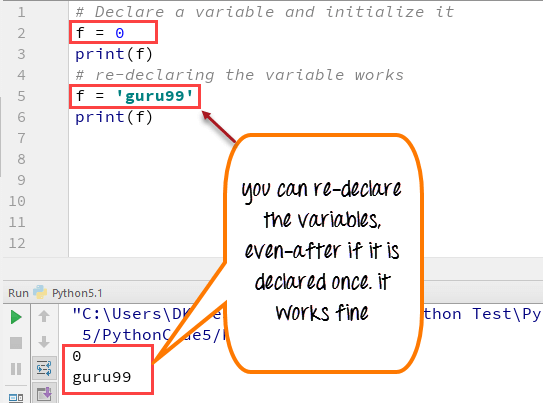Python 2 Example

``````# Declare a variable and initialize it
f = 0
print f
# re-declaring the variable works
f = 'guru99'
print f``````

Python 3 Example

``````# Declare a variable and initialize it
f = 0
print(f)
# re-declaring the variable works
f = 'guru99'
print(f)``````

## Python String Concatenation and Variable

Let’s see whether you can concatenate different data types like string and number together. For example, we will concatenate “Guru” with the number “99”.

Unlike Java, which concatenates number with string without declaring number as string, while declaring variables in Python requires declaring the number as string otherwise it will show a TypeError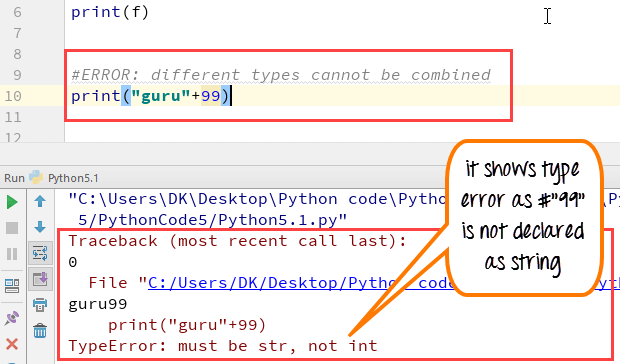For the following code, you will get undefined output –

``````a="Guru"
b = 99
print a+b``````

Once the integer is declared as string, it can concatenate both “Guru” + str(“99”)= “Guru99” in the output.

``````a="Guru"
b = 99
print(a+str(b))``````

## Python Variable Types: Local & Global

There are two types of variables in Python, Global variable and Local variable. When you want to use the same variable for rest of your program or module you declare it as a global variable, while if you want to use the variable in a specific function or method, you use a local variable while Python variable declaration.

Let’s understand this Python variable types with the difference between local and global variables in the below program.

1. Let us define variable in Python where the variable “f” is global in scope and is assigned value 101 which is printed in output
2. Variable f is again declared in function and assumes local scope. It is assigned value “I am learning Python.” which is printed out as an output. This Python declare variable is different from the global variable “f” defined earlier
3. Once the function call is over, the local variable f is destroyed. At line 12, when we again, print the value of “f” is it displays the value of global variable f=101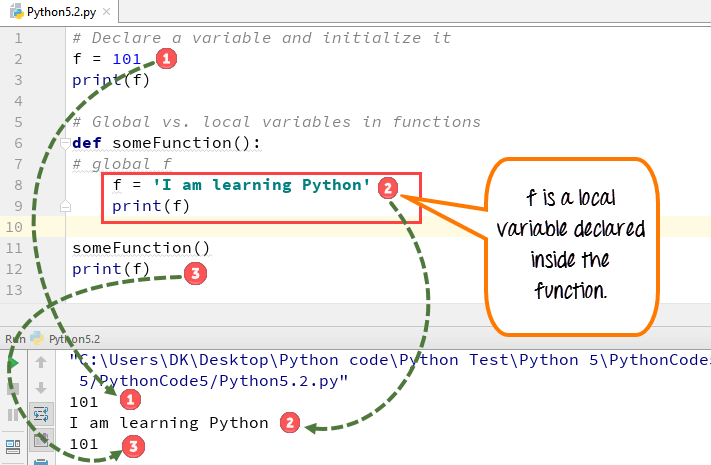Python 2 Example

``````# Declare a variable and initialize it
f = 101
print f
# Global vs. local variables in functions
def someFunction():
# global f
f = 'I am learning Python'
print f
someFunction()
print f``````

Python 3 Example

``````# Declare a variable and initialize it
f = 101
print(f)
# Global vs. local variables in functions
def someFunction():
# global f
f = 'I am learning Python'
print(f)
someFunction()
print(f)``````

While Python variable declaration using the keyword global, you can reference the global variable inside a function.

1. Variable “f” is global in scope and is assigned value 101 which is printed in output
2. Variable f is declared using the keyword global. This is NOT a local variable, but the same global variable declared earlier. Hence when we print its value, the output is 101

We changed the value of “f” inside the function. Once the function call is over, the changed value of the variable “f” persists. At line 12, when we again, print the value of “f” is it displays the value “changing global variable”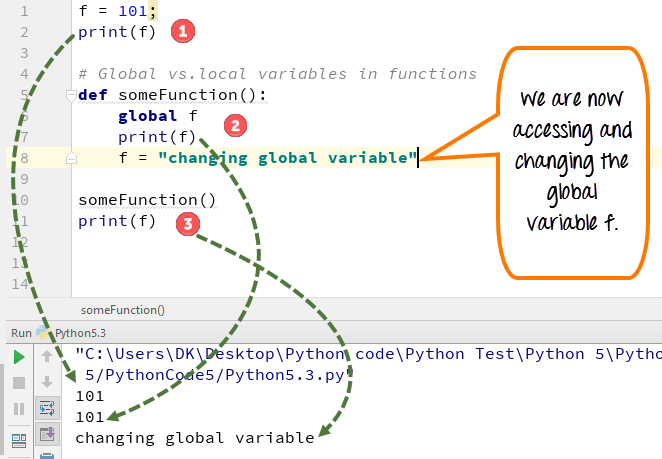Python 2 Example

``````f = 101;
print f
# Global vs.local variables in functions
def someFunction():
global f
print f
f = "changing global variable"
someFunction()
print f``````

Python 3 Example

``````f = 101;
print(f)
# Global vs.local variables in functions
def someFunction():
global f
print(f)
f = "changing global variable"
someFunction()
print(f)``````

## Delete a variable

You can also delete Python variables using the command del “variable name”.

In the below example of Python delete variable, we deleted variable f, and when we proceed to print it, we get error “variable name is not defined” which means you have deleted the variable.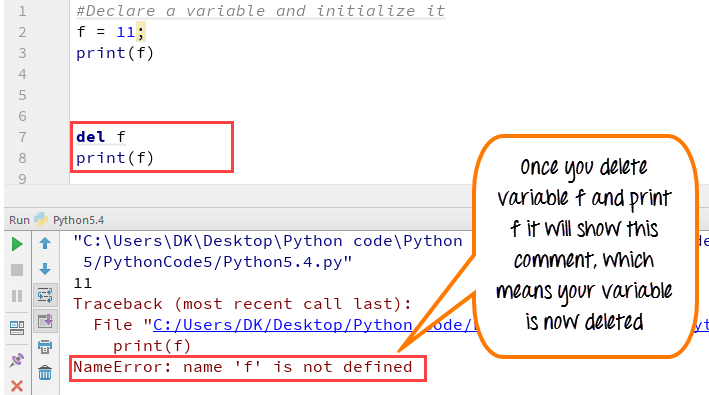Example of Python delete variable or Python clear variable :

``````f = 11;
print(f)
del f
print(f)``````

## Summary:

• Variables are referred to “envelop” or “buckets” where information can be maintained and referenced. Like any other programming language Python also uses a variable to store the information.
• Variables can be declared by any name or even alphabets like a, aa, abc, etc.
• Variables can be re-declared even after you have declared them for once
• Python constants can be understood as types of variables that hold the value which can not be changed. Usually Python constants are referenced from other files. Python define constant is declared in a new or separate file which contains functions, modules, etc.
• Types of variables in Python or Python variable types : Local & Global
• Declare local variable when you want to use it for current function
• Declare Global variable when you want to use the same variable for rest of the program

To delete a variable, it uses keyword “del”.

## A Beginner’s Guide To Python Variables

A variable is a fundamental concept in any programming language. It is a reserved memory location that stores and manipulates data. This tutorial on Python variables will help you learn more about what they are, the different data types of variables, the rules for naming variables in Python. You will also perform some basic operations on numbers and strings. We’ll use Jupyter Notebook to implement the Python codes.

Variables are entities of a program that holds a value. Here is an example of a variable:

x=100

In the below diagram, the box holds a value of 100 and is named as x. Therefore, the variable is x, and the data it holds is the value.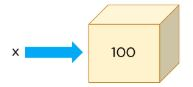The data type for a variable is the type of data it holds.

In the above example, x is holding 100, which is a number, and the data type of x is a number.

In Python, there are three types of numbers: Integer, Float, and Complex.

Integers are numbers without decimal points. Floats are numbers with decimal points. Complex numbers have real parts and imaginary parts.

Another data type that is very different from a number is called a string, which is a collection of characters.

Let’s see a variable with an integer data type:

``x=100``

To check the data type of x, use the type() function:

``type(x)``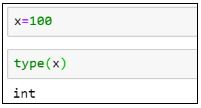Python allows you to assign variables while performing arithmetic operations.

``````x=654*6734
type(x)``````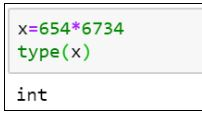To display the output of the variable, use the print() function.

``print(x) #It gives the product of the two numbers``

Now, let’s see an example of a floating-point number:

``````x=3.14
print(x)``````

type(x) #Here the type the variable is float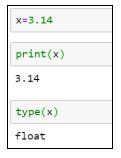Strings are declared within a single or double quote.

``````x=’Simplilearn’

print(x)

x=” Simplilearn.”

print(x)

type(x)``````

In all of the examples above, we only assigned a single value to the variables. Python has specific data types or objects that hold a collection of values, too. A Python List is one such example.

Here is an example of a list:

``````x=[14,67,9]

print(x)

type(x)``````

You can extract the values from the list using the index position method. In lists, the first element index position starts at zero, the second element at one, the third element at two, and so on.

To extract the first element from the list x:

``print(x)``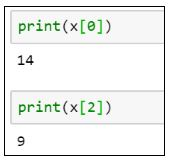To extract the third element from the list x:

``print(x)``

Lists are mutable objects, which means you can change the values in a list once they are declared.

``````x=70 #Reassigning the third element in the list to 70

print(x)``````

Earlier, the elements in the list had [14, 67, 9]. Now, they have [14, 67, 70].

Tuples are a type of Python object that holds a collection of value, which is ordered and immutable. Unlike a list that uses a square bracket, tuples use parentheses.

``````x=(4,8,6)

print(x)

type(x)``````

Similar to lists, tuples can also be extracted with the index position method.

``print(x) #Give the element present at index 1, i.e. 8``

If you want to change any value in a tuple, it will throw an error. Once you have stored the values in a variable for a tuple, it remains the same.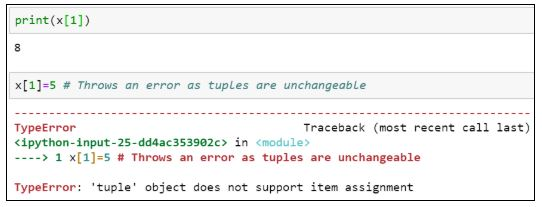When we deal with files, we need a variable that points to it, called file pointers. The advantage of having file pointers is that when you need to perform various operations on a file, instead of providing the file’s entire path location or name every time, you can assign it to a particular variable and use that instead.

Here is how you can assign a variable to a file:

``````x=open(‘C:/Users/Simplilearn/Downloads/JupyterNotebook.ipynb’,’r’)

type(x)``````

Suppose you want to assign values to multiple variables. Instead of having multiple lines of code for each variable, you can assign it in a single line of code.

``(x, y, z)=5, 10, 5``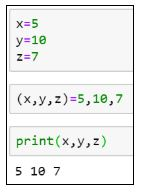The following line code results in an error because the number of values assigned doesn’t match with the number of variables declared.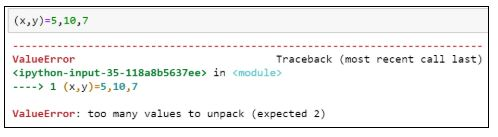If you want to assign the same value to multiple variables, use the following syntax:

``x=y=z=1``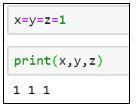Now, let's look at the various rules for naming a variable.

1. A variable name must begin with a letter of the alphabet or an underscore(_)

Example:

``````abc=100 #valid syntax

_abc=100 #valid syntax

3a=10 #invalid syntax

@abc=10 #invalid syntax``````

. The first character can be followed by letters, numbers or underscores.

Example:

``````a100=100 #valid

_a984_=100 #valid

a9967\$=100 #invalid

xyz-2=100 #invalid``````

Python variable names are case sensitive.

Example:

``````a100 is different from A100.

a100=100

A100=200``````

Reserved words cannot be used as variable names.

Example:

``````break, class, try, continue, while, if

break=10

class=5

try=100``````

Python is more effective and more comfortable to perform when you use arithmetic operations.

The following is an example of adding the values of two variables and storing them in a third variable:

``````x=20

y=10

result=x+y

print(result)``````

Similarly, we can perform subtraction as well.

``````result=x-y

print(result)``````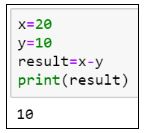Additionally, to perform multiplication and division, try the following lines of code:

``````result=x*y

print(result)

result=x/y

print(result)``````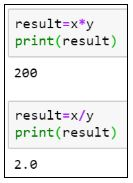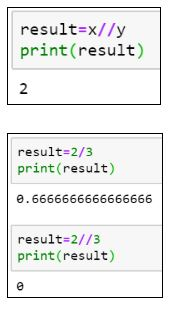As you can see, in the case of division, the result is not an integer, but a float value. To get the result of the division in integers, use “//”the integer division.

The division of two numbers gives you the quotient. To get the remainder, use the modulo (%) operator.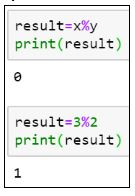Now that we know how to perform arithmetic operations on numbers let us look at some operations that can be performed on string variables.

``var = ‘Simplilearn’``

You can extract each character from the variable using the index position. Similar to lists and tuples, the first element position starts at index zero, the second element index at one, and so on.

``````print(var) #Gives the character at index 0, i.e. S

print(var) #Gives the character at index 4, i.e. l``````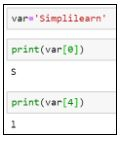If you want to extract a range of characters from the string variable, you can use a colon (:) and provide the range between the ones you want to receive values from. The last index is always excluded. Therefore, you should always provide one plus the number of characters you want to fetch.

``print(var[0:3]) #This will extract the first three characters from zero, first, and second index.``

The same operation can be performed by excluding the starting index.

``print(var[:3])``The following example prints the values from the fifth location until the end of the string.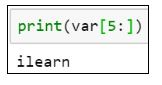Let’s see what happens when you try to print the following:

``print(var[0:20]) #Prints the entire string, although the string does not have 20 characters.``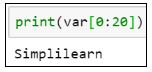To print the length of a string, use the len() function.

``len(var)``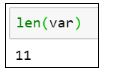Let’s see how you can extract characters from two strings and generate a new string.

``````var1 = “It’s Sunday”

var2 = “Have a great day”``````

The new string should say, “It’s a great Sunday” and be stored in var3.

``````var3 = var1[:5] + var2[5:13] + var1[5:]

print(var3)``````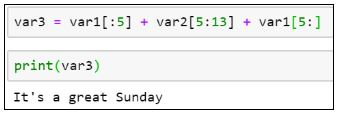Get prepared for your next career as a professional Python programmer with the Python Certification Training Course. Click to enroll now!

## Conclusion

I hope this blog helped you learn the concepts of Python variables. After reading this blog, you may have learned more about what a variable is, rules for declaring a variable, how to perform arithmetic operations on variables, and how to extract elements from numeric and string variables using the index position.

#python #programming

## Buddha Community1626775355

## Why use Python for Software Development

No programming language is pretty much as diverse as Python. It enables building cutting edge applications effortlessly. Developers are as yet investigating the full capability of end-to-end Python development services in various areas.

By areas, we mean FinTech, HealthTech, InsureTech, Cybersecurity, and that's just the beginning. These are New Economy areas, and Python has the ability to serve every one of them. The vast majority of them require massive computational abilities. Python's code is dynamic and powerful - equipped for taking care of the heavy traffic and substantial algorithmic capacities.

Programming advancement is multidimensional today. Endeavor programming requires an intelligent application with AI and ML capacities. Shopper based applications require information examination to convey a superior client experience. Netflix, Trello, and Amazon are genuine instances of such applications. Python assists with building them effortlessly.

## 5 Reasons to Utilize Python for Programming Web Apps

Python can do such numerous things that developers can't discover enough reasons to admire it. Python application development isn't restricted to web and enterprise applications. It is exceptionally adaptable and superb for a wide range of uses.

Robust frameworks

Python is known for its tools and frameworks. There's a structure for everything. Django is helpful for building web applications, venture applications, logical applications, and mathematical processing. Flask is another web improvement framework with no conditions.

Web2Py, CherryPy, and Falcon offer incredible capabilities to customize Python development services. A large portion of them are open-source frameworks that allow quick turn of events.

Python has an improved sentence structure - one that is like the English language. New engineers for Python can undoubtedly understand where they stand in the development process. The simplicity of composing allows quick application building.

The motivation behind building Python, as said by its maker Guido Van Rossum, was to empower even beginner engineers to comprehend the programming language. The simple coding likewise permits developers to roll out speedy improvements without getting confused by pointless subtleties.

Utilized by the best

Alright - Python isn't simply one more programming language. It should have something, which is the reason the business giants use it. Furthermore, that too for different purposes. Developers at Google use Python to assemble framework organization systems, parallel information pusher, code audit, testing and QA, and substantially more. Netflix utilizes Python web development services for its recommendation algorithm and media player.

Massive community support

Python has a steadily developing community that offers enormous help. From amateurs to specialists, there's everybody. There are a lot of instructional exercises, documentation, and guides accessible for Python web development solutions.

Today, numerous universities start with Python, adding to the quantity of individuals in the community. Frequently, Python designers team up on various tasks and help each other with algorithmic, utilitarian, and application critical thinking.

Progressive applications

Python is the greatest supporter of data science, Machine Learning, and Artificial Intelligence at any enterprise software development company. Its utilization cases in cutting edge applications are the most compelling motivation for its prosperity. Python is the second most well known tool after R for data analytics.

The simplicity of getting sorted out, overseeing, and visualizing information through unique libraries makes it ideal for data based applications. TensorFlow for neural networks and OpenCV for computer vision are two of Python's most well known use cases for Machine learning applications.

### Summary

Thinking about the advances in programming and innovation, Python is a YES for an assorted scope of utilizations. Game development, web application development services, GUI advancement, ML and AI improvement, Enterprise and customer applications - every one of them uses Python to its full potential.

The disadvantages of Python web improvement arrangements are regularly disregarded by developers and organizations because of the advantages it gives. They focus on quality over speed and performance over blunders. That is the reason it's a good idea to utilize Python for building the applications of the future.

#python development services #python development company #python app development #python development #python in web development #python software development1593156510

## Basic Data Types in Python | Python Web Development For Beginners

At the end of 2019, Python is one of the fastest-growing programming languages. More than 10% of developers have opted for Python development.

In the programming world, Data types play an important role. Each Variable is stored in different data types and responsible for various functions. Python had two different objects, and They are mutable and immutable objects.

I Mutable objects

II Immutable objects

III Built-in data types in Python

## Mutable objects

The Size and declared value and its sequence of the object can able to be modified called mutable objects.

Mutable Data Types are list, dict, set, byte array

## Immutable objects

The Size and declared value and its sequence of the object can able to be modified.

Immutable data types are int, float, complex, String, tuples, bytes, and frozen sets.

id() and type() is used to know the Identity and data type of the object

a**=25+**85j

type**(a)**

output**:<class’complex’>**

b**={1:10,2:“Pinky”****}**

id**(b)**

output**:**238989244168

## Built-in data types in Python

a**=str(“Hello python world”)****#str**

b**=int(18)****#int**

c**=float(20482.5)****#float**

d**=complex(5+85j)****#complex**

e**=list((“python”,“fast”,“growing”,“in”,2018))****#list**

f**=tuple((“python”,“easy”,“learning”))****#tuple**

g**=range(10)****#range**

h**=dict(name=“Vidu”,age=36)****#dict**

i**=set((“python”,“fast”,“growing”,“in”,2018))****#set**

j**=frozenset((“python”,“fast”,“growing”,“in”,2018))****#frozenset**

k**=bool(18)****#bool**

l**=bytes(8)****#bytes**

m**=bytearray(8)****#bytearray**

n**=memoryview(bytes(18))****#memoryview**

## Numbers (int,Float,Complex)

Numbers are stored in numeric Types. when a number is assigned to a variable, Python creates Number objects.

#signed interger

age**=**18

print**(age)**

Output**:**18

Python supports 3 types of numeric data.

int (signed integers like 20, 2, 225, etc.)

float (float is used to store floating-point numbers like 9.8, 3.1444, 89.52, etc.)

complex (complex numbers like 8.94j, 4.0 + 7.3j, etc.)

A complex number contains an ordered pair, i.e., a + ib where a and b denote the real and imaginary parts respectively).

## String

The string can be represented as the sequence of characters in the quotation marks. In python, to define strings we can use single, double, or triple quotes.

# String Handling

‘Hello Python’

#single (') Quoted String

“Hello Python”

# Double (") Quoted String

“”“Hello Python”“”

‘’‘Hello Python’‘’

# triple (‘’') (“”") Quoted String

In python, string handling is a straightforward task, and python provides various built-in functions and operators for representing strings.

The operator “+” is used to concatenate strings and “*” is used to repeat the string.

“Hello”+“python”

output**:****‘Hello python’**

"python "*****2

'Output : Python python ’

#python web development #data types in python #list of all python data types #python data types #python datatypes #python types #python variable type1624934525

## Python I: Data Types and Operators, variable assignment, and print()

### Learn the Python basics so that you can use it for your data science projects.

This blog is part of a series of tutorials called Data in Day. Follow these tutorials to create your first end-to-end data science project in just one day. This is a fun easy project that will teach you the basics of setting up your computer for a data science project and introduce you to some of the most popular tools available. It is a great way to get acquainted with the data science workflow.

Created by Dutch programmer Guido van Rossum at Centrum Wiskunde & Informatica, Python made its debut in 1991. Over thirty years it has gained popularity earned a reputation of being the “Swiss army knife of programming languages.” Here are a few reasons why:

In emerging fields like data science, artificial intelligence, and machine learning, a robust community, plenty of packages, paradigm flexibility, and syntactical simplicity, allow beginners and professionals to focus on insights and innovation.

#python3 #variables-in-python #data-types-in-python #operators-in-python #python #python i: data types and operators, variable assignment, and print()1602968400

## Python Tricks Every Developer Should Know

Python is awesome, it’s one of the easiest languages with simple and intuitive syntax but wait, have you ever thought that there might ways to write your python code simpler?

In this tutorial, you’re going to learn a variety of Python tricks that you can use to write your Python code in a more readable and efficient way like a pro.

### Let’s get started

Swapping value in Python

Instead of creating a temporary variable to hold the value of the one while swapping, you can do this instead

``````>>> FirstName = "kalebu"
>>> LastName = "Jordan"
>>> FirstName, LastName = LastName, FirstName
>>> print(FirstName, LastName)
('Jordan', 'kalebu')
``````

#python #python-programming #python3 #python-tutorials #learn-python #python-tips #python-skills #python-development1602666000

## How to Remove all Duplicate Files on your Drive via Python

Today you’re going to learn how to use Python programming in a way that can ultimately save a lot of space on your drive by removing all the duplicates.

### Intro

In many situations you may find yourself having duplicates files on your disk and but when it comes to tracking and checking them manually it can tedious.

Heres a solution

But How do we do it?

If we were to read the whole file and then compare it to the rest of the files recursively through the given directory it will take a very long time, then how do we do it?

The answer is hashing, with hashing can generate a given string of letters and numbers which act as the identity of a given file and if we find any other file with the same identity we gonna delete it.There’s a variety of hashing algorithms out there such as

• md5
• sha1
• sha224, sha256, sha384 and sha512

#python-programming #python-tutorials #learn-python #python-project #python3 #python #python-skills #python-tips